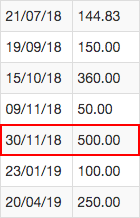# CAST NUMERIC from SQLite

Hi there, double question today. This new one about the DOUBLE values !

Theoretically an extremely simple question but I found no examples that help me with this.``````Var sql As String = "SELECT Amount FROM Table WHERE Amount = (SELECT MAX(CAST(Amount AS NUMERIC)) FROM Table)"
``````

Substantially I’m trying to retrieve the MAX Value from a DOUBLE values column but using the current expression I’m just getting 0.00 while it should be 500.00. I’m not sure what the mistake is.

If all you’re trying to do is get 500.00 this SQL should do the trick:

``````SELECT MAX(Amount) FROM Table;
``````

Reference material:
https://www.w3schools.com/sql/sql_min_max.asp

1 Like

It’s funny because the value is changed now but I still don’t get the MAX one.

Ok, I maybe understand now the problem is with the way I’m managing it in Xojo:

``````Var Max_Amount As Double = rs.ColumnAt(0).DoubleValue
``````

ORDER BY DESC right?

Looks like the problem here is that you dont understad how the aggregate functions work in SQL. Thos return a SINGLE value. That is, a SINGLE column with a SINGLE row and that column IS NOT one of the columns of your table.

Could try:

``````SELECT MAX(Amount) As maximum FROM Table;
``````

That should be usable using:

``rs.Column("maximum").DoubleValue``
1 Like

Right, I’ve been using GROUP + ORDER BY for such a long time before that I just copied it and didn’t think about. However a skimpy pratical example like @DerkJ gently provided would have been surely more useful to me and many others that could be in the same situation of mine, don’t you think? Personally I think this space not only for experts but particularly for ignorant people like me, quite logical after all.

Thank you @DerkJ, for some reasons I cannot figure out it just returns the first value of the column but I appreciate the example, now I better understand where I must focus on.

You can get this:

``````SELECT MAX(amount) FROM Table;
``````

By doing this:

``````rs.Column("MAX(amount)").DoubleValue
``````

But that reads a little odd.

1 Like

I think I got it, that one you see is not the complete table and now I understand the problem: the first value that’s a DOUBLE equal to 95.21 are always returned as MAX value just because when compared it is 9521 > 500.

The only logical explanation.

It does not explain what happens to 144.83 but I cannot see any other reason.

Next try:

``SELECT Amount FROM Table WHERE Amount = (SELECT MAX(Amount) FROM Table LIMIT 1)``

95.21``````// Collect Top_Reward
``````

If App.DB.Connect Then

Var sql As String = “SELECT Amount FROM Table WHERE Amount = (SELECT MAX(Amount) FROM Table LIMIT 1)”

Var rs As RowSet = App.DB.SelectSQL(sql)

If rs Is Nil Then

``````Return False
``````

` Else`

``````// Set Values

Var Max_Amount As Double = rs.Column("Amount").DoubleValue

mTop_Amount = Max_Amount

MessageBox(Max_Amount.ToString)

End If

End If``````

Did you store the values as doubles or as strings ?

When i try:

``````SELECT salary FROM test WHERE salary = (SELECT MAX(salary) FROM test LIMIT 1)
``````

i get back 500.0 with test data:

``````INSERT INTO test (salary, name) VALUES(500.5, 'high value')
``````

and

``````INSERT INTO test (salary, name) VALUES(144.0, 'lower value')
``````

It works flawless. with a test table:

``````CREATE TABLE "test" (
"id"	INTEGER,
"salary"	REAL DEFAULT 0.0,
"name"	TEXT,
PRIMARY KEY("id")
);``````
1 Like

You are right, I store it as Double but I forgot to change the Table from which I pick it.

Big Sorry!

1 Like

Oh well, it happens…1 Like

Thank you for the comprehension. I hate you have been wasted your time on such nonsense.

As long as you approach it with the attitude to learn, we’re all happy to help here1 Like

Frankly I don’t like the idea of asking for people to do my homework, you know… As ignorant I see a lot of topics all over the web and you well know what I mean.

Sometimes I’m just forced to because this is not my profession, anyway as I said in my previous topic today I’m happy with Xojo forum, the gentle effort most of proffesionist put on it everyday.